# Smart Sim – binary addition

### Smart Sim Tutorial

SmartSim is a free, and open source, digital logic circuit design and simulation package for anyone interested in electronics, and as such its a valuable teaching aid.

It was created by the then 16-year old sixth form student Ashley Newson from Oxford, UK. Its based using the Vala object-oriented programming language, the GTK+ and Cairo graphics libraries, and is available on both Linux and Windows.

If you own a Raspberry Pi then SmartSim comes pre installed, but it can also be downloaded directly from Smartsim.org.uk. As well as the App, you will also need the SmartSim manual. The manual is concise and well written, and includes a tutorial to get you started. It is a natural and obvious complement for the Raspberry Pi.

The user interface is also quite straight forward, The only thing I personally found a bit quirky, was the component orientation feature.

I’ll leave you to work through the tutorial in the manual, and pick up where that leaves off. For this tutorial I’ll work through the binary adder circuit, (one of the examples included on the site), to fill in some of the techniques you’ll need to design your own circuits.

### Starting SmartSim – A Half Adder Tutorial

Open SmartSim, and under the file menu click new project, then click on new component. The first circuit we will build first is an Exclusive OR (XOR of short), to illustrate the b>hierarchical feature of SmartSim. The component layout is show below, so connect up the circuit as shown. I’m assuming at this stage, you are now familiar with the basic interface. If not then I recommend you work through the example in the user manual. It’s actually the same circuit shown here.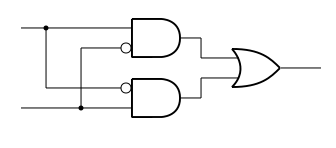From the file menu click on Save the component as and save it as xor.ssc.

The Exclusive OR (XOR) circuit actually consists of two AND gates and one OR gate. The AND gate is the one that looks like a D. (An easy way to remember which is which, is remember the D and AND. Each of the AND gates has one of its inputs negated (or inverted. The circle on the input indicates that it is negated. This is third type of gate that actually make up the 3 basic building blocks of all digital circuits, including the computer you are using now! The full symbol for a not gate is shown below, but usually it gets abbreviated to a circle on either an input or output. You can see from the symbol it is a buffer (amplifier) with its output inverted, i.e. if the input is true (or logic 1) then the output is false (or logic 0), e.g. NOT true. Likewise if the input is false then the the output is NOT false (e.g. true). The exclusive OR circuit is so common it to has its own special symbol (shown below).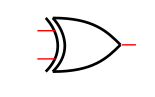### Truth tables

Why is this called Exclusive OR, and not just OR? To explain we’ll make use of a truth table. For every possible input condition a truth table simply shows the corresponding output. Lets finish off our custom XOR circuit by adding labels to the inputs and output as shown.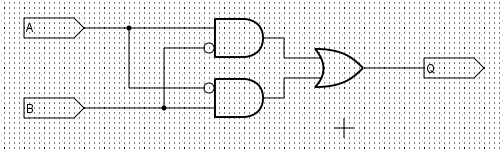Select the interface tag, and from the end of a wire drag the mouse and release it to get the interface tag screen (below).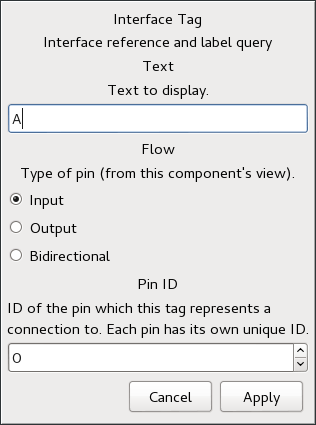Give the tag a name, in this case A and don’t forget to select the correct type of pin; again in this case input. Repeat this for the other two pins and save the circuit (as xor.ssc) again.

#### Creating a custom component

Under the Component tab, select the, set as root option, then select the customise option; to get the customise screen. If this step seems familiar, its actually the same as the SmartSim tutorial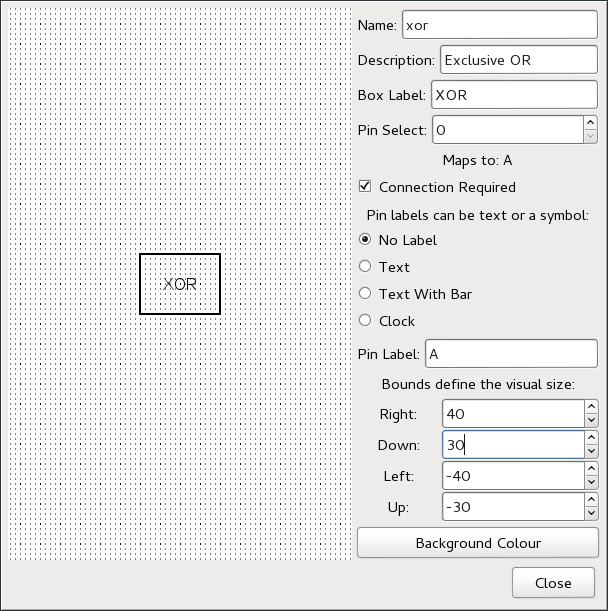Give the custom circuit a name, and complete the description and box label. Next fill in the box dimensions. You can choose what values you like, to suit the particular component. Lastly for each pin, (in this case) select text and click on a position outside one of the four corners of the box, to locate the pin. Repeat this step for the remaining pins, then when you have finished, close the screen, and from the file menu, save the component.

Now you are ready to make use of the new component. From the file menu, select new component and from the user icon, select the circuit you have just created. Connect up two toggles (for the inputs) and a reader for the output. From the component menu select the component as root and then from the run menu, check the circuit validity. If everything is OK select run from the run menu to start the simulation.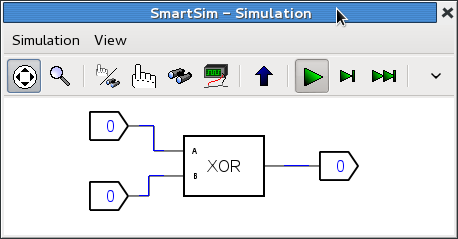Now we’ll (manually) create the truth table for this circuit. Select the context icon (the hand and binoculars). The first entry in the truth is 0, when A = 0 and B = 0. Now change each input in turn and verify the output is as shown below. Incidentally the inputs A and B are the binary equivalent of the decimal number in the left hand column.Exclusive OR
num A B Q
0 0 0 0
1 0 1 1
2 1 0 1
3 1 1 0

Conventionally 0 = false and 1 equals true, so we can express the truth table for exclusive OR in words as follows.

The output (Q) is true when A is true (but NOT B) OR when B is true (but NOT A).

This is usually what we mean in everyday speech. Contrast this with the true table for (logical) OR, below. Now you can see that the output is true if A OR B is true; and of course if they are both true then the output is still true.

OR
num A B Q
0 0 0 0
1 0 1 1
2 1 0 1
3 1 1 1

Just for the sake of completeness, here is the truth table for AND. You can see from this table the output is only true if A AND B is true

AND
num A B Q
0 0 0 0
1 0 1 0
2 1 0 0
3 1 1 1

### Building a half adder

So what has this to do with addition?. In the previous example, we started with a circuit and deduced its truth table. Froam a design point of view we start with the truth table and need to deduce an equivalent circuit. That’s what we are going to do next, but first a refresher on binary arithmetic.

If we add 0 + 0 = 0 the answer is still nought. Also adding 1 + 0 = 1, or for that matter 0 + 1 = 1. But what if we add (binary) 1 + 1 = 10. The answer not (decimal) ten but binary 0 carry 1; because binary arithmetic only has the digits 0 and 1. It’s analogous to decimal arithmetic when we add 9 + 1 = 10. I.e. the answer is 0 carry 1. (though in everyday speech we would say ten). That is because we only have ten symbols (0 to 9 ) in decimal arithmetic, so the position of these symbols is important. Each time we move one position left we multiply by the base (10 in this case).

Decimal weights
1 x 10 x 10 x 10 1 x 10 x 10 1 x 10 1
thousand hundred ten unit

The rules of binary arithmetic are exactly the same, the only thing that’s changed is the base (now 2). So what is the largest number we can express with just four (binary) digits, i.e. 1111. That is 1 eight + 1 four + 1 two + 1 one. which gives (decimal) 15.

Binary weights
1 x 2 x 2 x 2 1 x 2 x 2 1 x 2 1
eight four two unit

OK with that under our belts we can now go back and construct the truth table for a half adder, to add two binary digits (bits) together. From the table you can see we now have two inputs and two outputs, one for the sum and the other for the carry bit. Now I hope you can see from this table that the sum column is the same as our old friend Exclusive OR, while the carry column is the same as the truth table for an AND gate.

Half Adder
A B Sum Carry
0 0 0 0
0 1 1 0
1 0 1 0
1 1 0 1

The completed half adder circuit is shown below. Add the tags to the input and outputs and save this component. Also save the project, which should now include both the half adder and the custom XOR circuit in its list of user components.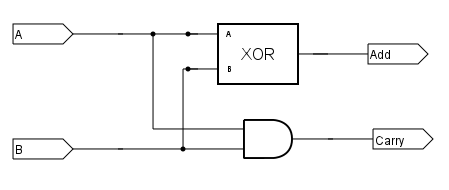### Binary Full Adder

The half adder can be expanded to a full adder by the inclusion
of an input carry. The truth table and logical equivalent are
shown below. The first half of the truth table has been arranged
so that it is the same as the half adder (i.e. the carry in bit is zero). The bottom half of the table shows the effect of adding the additional carry bit in.

### Full Adder – Truth table and symbolic representation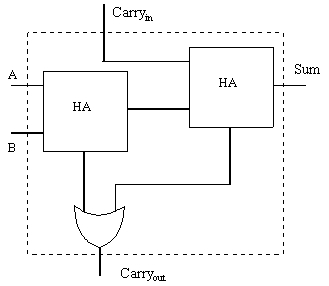A B Carryin Sum Carryout 0 0 0 0 0 0 1 0 1 0 1 0 0 1 0 1 1 0 0 1 0 0 1 1 0 0 1 1 0 1 1 0 1 0 1 1 1 1 1 1

The first half adder adds the bits (A + B) together, then the sum of these values is added to the carry bit in the second half adder. Again I hope you can see that the carryout from this if a carry from the first HA, OR a carry from the second.

Create a new component and now build the full adder circuit shown, using two HA circuits you have already built. Set this new circuit as root and save it as a Full adder.

### 4 bit binary addition

Finally by connecting 4 Full adders together its now possible to demonstrate 4 bit binary addition. The least significant bit (LSB) is shown on the right, and has the carryin bit set to zero.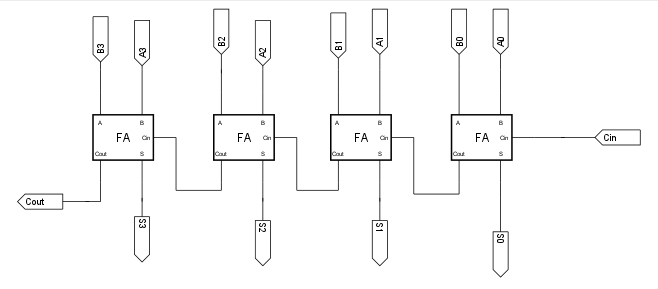Using the 4 bit adder, connect the circuit up as shown to test the circuit interactively. Use the toggles to set the two 4 bit binary numbers. The output readers show the binary result, while the displays show the hexadecimal equivalent (base 16).

Hexadecimal numbers use the regular digits 0 – 9 then letters A,B,C,D,E,F to represent the hex equivalent of (decimal) 10 – 15. So the example shows the addition of hex 1 + F (decimal 15) = hex 10 (or decimal 16).

Hexadecimal weights
1 x 16 x 16 x 16 1 x 16 x 16 1 x 16 1
4096 256 16 unit

Clearly when writing about decimal numbers, and numbers of a different base, on the same page can be confusing. To clarify this, by convention, the number base is added as a subscript/ E.g.

```    116 + F16 = 1016

is equivalent to

110 + 1510 = 1610
```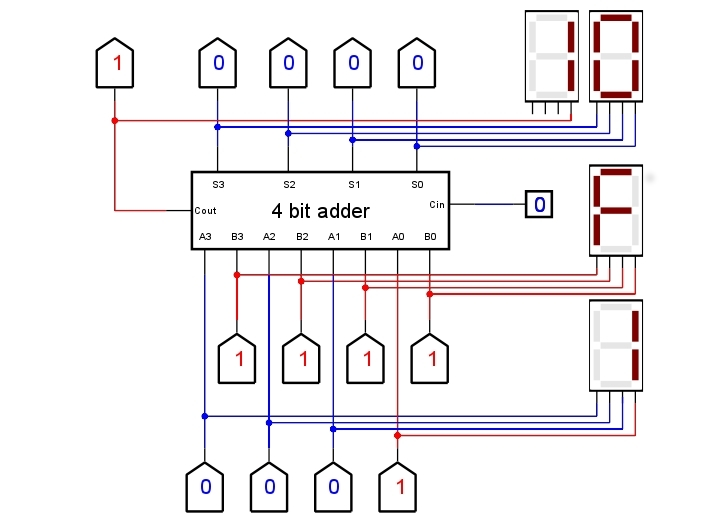This has necessarily been a brief introduction to SmartSim, as an aid to teaching digital electronics. Even so its been possible to show how a computer can, using different number bases, do binary and hexadecimal arithmetic, and introduce the concept of truth tables, to relate input and outputs.